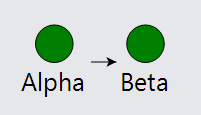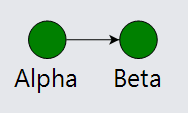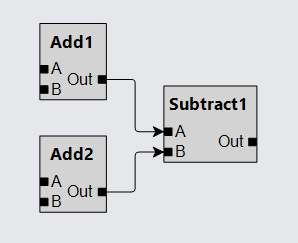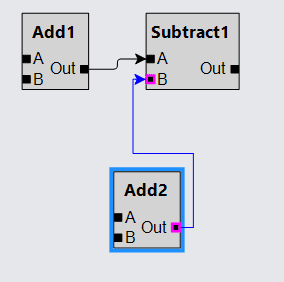# Ports in Nodes

Although you have some control over where links will connect to a node (at a particular spot, along one or more sides, or at the intersection with the edge), there are times when you want to have different logical and graphical places at which links should connect. The elements at which a link may connect are called ports. There may be any number of ports in a node. By default there is just one port, the whole node, which results in the effect of having the whole node act as the port, as you have seen in all of the previous examples.

To declare that a particular element in a Node is a port, set the GraphObject.PortId property to a string. Note that the port-or-link-related properties may apply to any element in the visual tree of the node, which is why they are properties of GraphObject rather than Node.

Port-like GraphObjects can only be in Nodes or Groups, not in Links or Adornments or simple Parts. So there is no reason to try to set GraphObject.PortId on any object in a Link.

See samples that make use of ports in the samples index.

## Single Ports

In many situations you want to consider links logically related to the node as a whole but you don't want links connecting to the whole node. In this case each node has only one port, but you do not want the whole node to act as the one port.

For example, consider how links connect to the nodes when the whole node is acting as a port in one common manner. The GraphObject.FromSpot and GraphObject.ToSpot are at the middles of the sides. Because the height of the whole node includes the text label, the middle of the side is not the middle of the "icon", which in this case is a circle.

``````
diagram.NodeTemplate =
new Node("Vertical") {
FromSpot = Spot.Right, ToSpot = Spot.Left  // port properties on the node
}
new Shape("Circle") { Width = 30, Fill = "green" },
new TextBlock { Font = new Font("Segoe UI", 20) }
.Bind("Text", "Key")
);

diagram.Model =
new MyModel {
NodeDataSource = new List<NodeData> {
new NodeData { Key = "Alpha" },
new NodeData { Key = "Beta" }
},
new LinkData { From = "Alpha", To = "Beta" }
}
};
``````This appearance does not look or behave quite right. Really we want links to connect to the circular Shape.

If you want a particular element to act as the port rather than the whole node, just set its GraphObject.PortId to the empty string. The empty string is the name of the default port.

In this example, we set GraphObject.PortId on the circular shape. Note that we move the other port-related properties, such as the port spots, to that object too.

``````
diagram.NodeTemplate =
new Node("Vertical")
new Shape("Circle") {
Width = 30, Fill = "green",
PortId = "",  // now the Shape is the port, not the whole Node
FromSpot = Spot.Right, ToSpot = Spot.Left  // port properties go on the port!
},
new TextBlock { Font = new Font("Segoe UI", 20) }
.Bind("Text", "Key")
);

diagram.Model =
new MyModel {
NodeDataSource = new List<NodeData> {
new NodeData { Key = "Alpha" },
new NodeData { Key = "Beta" }
},
new LinkData { From = "Alpha", To = "Beta" }
}
};
``````Notice how the links nicely connect the circular shapes by ignoring the text labels.

## General Ports

It is also common to have diagrams where you want more than one port in a node. The number of ports might even vary dynamically.

Normally a GraphLinksModel assumes that there is no need to recognize port information on link data. If you want to support port identifiers on link data, you need to set GraphLinksModel.LinkToPortIdProperty and GraphLinksModel.LinkFromPortIdProperty to be the names of the link data properties. If you do not set these properties, all port identifiers are assumed to be the empty string, which is the name of the one default port for a node.

``````
diagram.NodeTemplate =
new Node("Auto")
new Shape { Fill = "lightgray" },
new Panel("Table")
new ColumnDefinition { Column = 0, Alignment = Spot.Left },
new ColumnDefinition { Column = 2, Alignment = Spot.Right }
)
new TextBlock {  // the node title
Column = 0, Row = 0, ColumnSpan = 3, Alignment = Spot.Center,
Font = new Font("Segoe UI", 14, FontWeight.Bold), Margin = new Margin(4, 2)
}
.Bind("Text", "Key"),
new Panel("Horizontal") { Column = 0, Row = 1 }
// the "A" port
new Shape { Width = 6, Height = 6, PortId = "A", ToSpot = Spot.Left },
new TextBlock("A")
),
new Panel("Horizontal") { Column = 0, Row = 2 }
// the "B" port
new Shape { Width = 6, Height = 6, PortId = "A", ToSpot = Spot.Left },
new TextBlock("B")
),
new Panel("Horizontal") { Column = 2, Row = 1, RowSpan = 2 }
// the "A" port
new TextBlock("Out"),
new Shape { Width = 6, Height = 6, PortId = "Out", FromSpot = Spot.Right }
)
)
);

new Shape(),
new Shape { ToArrow = "Standard" }
);

diagram.Layout = new LayeredDigraphLayout { ColumnSpacing = 10 };

diagram.Model =
new MyModel {
LinkFromPortIdProperty = "FromPort",  // required information:
LinkToPortIdProperty = "ToPort",      // identifies data property names
NodeDataSource = new List<NodeData> {
new NodeData { Key = "Add1" },
new NodeData { Key = "Add2" },
new NodeData { Key = "Subtract1" }
},
new LinkData { From = "Add1", FromPort = "Out", To = "Subtract1", ToPort = "A" },
new LinkData { From = "Add2", FromPort = "Out", To = "Subtract1", ToPort = "B" }
}
};
``````Setting either or both of the GraphObject.FromLinkable and GraphObject.ToLinkable properties to true allows users to interactively draw new links between ports.

To draw a new link, mouse down on an "Out" port, move (drag) to nearby an input port, and then mouse-up to complete the link.

``````
diagram.NodeTemplate =
new Node("Auto")
new Shape { Fill = "lightgray" },
new Panel("Table")
new ColumnDefinition { Column = 0, Alignment = Spot.Left },
new ColumnDefinition { Column = 2, Alignment = Spot.Right }
)
new TextBlock {  // the node title
Column = 0, Row = 0, ColumnSpan = 3, Alignment = Spot.Center,
Font = new Font("Segoe UI", 14, FontWeight.Bold), Margin = new Margin(4, 2)
}
.Bind("Text", "Key"),
new Panel("Horizontal") { Column = 0, Row = 1 }
// the "A" port
new Shape {
Width = 6, Height = 6, PortId = "A", ToSpot = Spot.Left,
},
new TextBlock("A")
),
new Panel("Horizontal") { Column = 0, Row = 2 }
// the "B" port
new Shape {
Width = 6, Height = 6, PortId = "A", ToSpot = Spot.Left,
},
new TextBlock("B")
),
new Panel("Horizontal") { Column = 2, Row = 1, RowSpan = 2 }
// the "A" port
new TextBlock("Out"),
new Shape {
Width = 6, Height = 6, PortId = "Out", FromSpot = Spot.Right,
}
)
)
);

new Shape(),
new Shape { ToArrow = "Standard" }
);

diagram.Layout = new LayeredDigraphLayout { ColumnSpacing = 10 };

diagram.Model =
new MyModel {
LinkFromPortIdProperty = "FromPort",  // required information:
LinkToPortIdProperty = "ToPort",      // identifies data property names
NodeDataSource = new List<NodeData> {
new NodeData { Key = "Add1" },
new NodeData { Key = "Add2" },
new NodeData { Key = "Subtract1" }
},